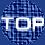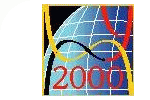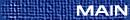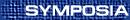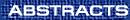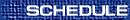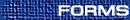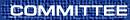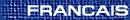Partial Differential Equations / Équations aux dérivées partielles
(Pengfei Guan, Organizer)

STEPHEN ANCO, Brock

DAN BURNS, Department of Mathematics, University of Michigan, Ann Arbor Michigan  48109-1109, USA
Algebraicization of entire Grauert tubes

(This is joint work with Raúl Aguilar of Tufts University.) A Grauert tube is a complex manifold (structure) defined on a neighborhood of the zero-section in the (co-)tangent bundle of an underlying real analytic Riemannian manifold. The radius of existence, or convergence, of this structure is the maximal radius in terms of the Riemannian length function on tangent vectors on which the structure can be defined. This radius is controled by subtle geometric and analytic features of the Riemannian metric. Examples of entire tubes (tubes with infinite radius) are rare, and we are trying to understand, perhaps classify, this phenomenon. We prove two theorems in this direction. First, we show that an entire Grauert tube is, in a natural way, an affine algebraic variety. Second, for a tube of complex dimension two, we show that this affine variety is either the product of two punctured planes (for a flat metric on a real torus), or is the affine quadric in complex three space, if the underlying real surface is the two sphere. The first result relies heavily on Demailly's theory of Monge-Ampère measures and the fact that the length function on tangent vectors is a solution of the homogeneous complex Monge-Ampère equation, while the second follows from the classical theory of minimal models for projective algebraic surfaces.

S. CHANILLO, Rutgers

WALTER CRAIG, Brown

NASSIF GHOUSSOUB, University of British Columbia, British Columbia
To be announced

ZHENG-CHAO HAN, Department of Mathematics, Rutgers University, Piscataway, New Jersey  08854-8019, USA
Existence and compactness results of the Yamabe problem with boundary

Let (Mn,g0) be a compact Riemannian manifold with boundary M. We consider the problem of looking for a metric g on M conformal to g0 and having constant scalar curvature c1 on M and constant mean curvature c2 on M. This is a generalization of the Yamabe problem to manifolds with boundary. Previous existence results on this problem were obtained by J. Escobar in the case c1 = 0 and c2 appropriately normalized, and in the case c2 = 0 or near 0 and c1 appropriately normalized. We will present new existence and apriori estimates of such metrics for any prescribed c2(after c1 has been appropriately normalized).

VICTOR IVRII, Department of Mathematics, University of Toronto, Toronto, Ontario  M5S 3G3
Spectral asymptotics with sharp remainder estimates for operators with low regularity condition

Weyl conjecture holds for second-order operators with C1+s coefficients in domains with C1+s boundary. This and similar theorems, proven recently, are subject of my talk.

HOWARD JACOBOWITZ, Rutgers University, Camden, New Jersey  08102, USA
On the existence of elliptic pseudodifferential operators

Given two complex vector bundles E, F over a compact manifold M, we study the existence and classification of elliptic pseudodifferential operators from sections of E to sections of F. Using the Gysin sequence of the cosphere bundle of M, it is easy to see the necessary condition that ci(E) = ci(F) for i < m and cm(E) - cm(F) = k c where ci denote the i-th Chern class and c is the Euler characteristic of M. In the case of line bundles this condition is also sufficient. (This is joint work with Gerardo Mendoza of Temple University).

SONG-YING LI, University of California, Irvine, California, USA, and University of Maryland, Maryland, USA
Boundedness of the second derivatives of Green's operators on H1 and BMO

Let W be a bounded domain in Rn. Let A(x) = (ajk(x)) be an n×n symmetric matrix such that ajk Î L¥(W) and lIn £ A(x) £ L In for a.e. x Î W with 0 < l £ L < ¥. Let G [f] the Green operator be the solution operator of the Dirichlet problem of non-divergent form elliptic equation:

 n å j,k = 1 ajk(x) ¶2 u¶xj ¶xk = f in W and u = 0 on ¶W.
We give sufficient, and almost necessary conditions on the smoothness of ajk and W so that the second derivatives of the Green's operator (2i j G[f]) are bounded on H1(W) and bounded BMO(W).

XINAN MA, East China Normal University, China
To be announced

ROBERT MCCANN, Department of Mathematics, University of Toronto, Toronto, Ontario  M5S 3G3
Kinetic equilibration rates for granular media

This joint work with José Carillo and Cedric Villani provides an algebraic decay rate bounding the time required for velocities to equilibrate in a spatially homogeneous flow-through model representing the continuum limit of a gas of particles interacting through slightly inelastic collisions. The rate is obtained by reformulating the dynamical problem as the gradient flow of a convex energy on an infinite-dimensional Riemannian manifold. An abstract theory is developed for gradient flows which shows how degenerate convexity (or even non-convexity)-if uniformly controlled-will quantify contractivity of the flow.

HAYATO NAWA, Nagoya University, Chikusa-ku, Nagoya  464-8602, Japan
Blowup of solutions of a class of nonlinear Schrödinger equations

We consider the existence of singular solutions of the nonlinear Schrödinger equations with local interactions, and their asymptotic behavior.

DMITRY PELINOVSKY, University of Toronto and McMaster University
Degenerate rational solutions of the KP hierarchy

Rational solutions of the KP hierarchy are reviewed by means of the vertex operator methods. I will show how to construct and classify general rational solutions of the l-reduced and k-constrained KP hierarchies as well as of their generalized counterparts.

E. POLETSKY, Syracuse
To be announced

JOEL SMOLLER, Department of Mathematics, University of Michigan, Ann Arbor, Michigan  48109, USA
Non-formation of vacuum states for the compressible Navier-Stokes equations

Under general conditions on the initial data, we prove that for the compressible Navier-Stokes equations, if no vacuum is present at t = 0, then no vacuum is present at all t > 0.

(This is joint work with David Hoff.)

CATHERINE SULEM, Department of Mathematics, University of Toronto, Toronto, Ontario
Asymptotic stability of solitary waves for nonlinear Schrödinger equations

We investigate the long time behavior of solutions whose initial conditions are close to solitary waves. In particular, we compute explicitly the leading terms, determine the rate of convergence as well as the period of oscillations, and give uniform bounds on the reminders.

DANIEL TATARU, Northwestern University
On Strichartz type estimates and the nonlinear wave equation

We present some new estimates of Strichartz type for the wave equation with nonsmooth coefficients. These estimates can in turn be used to improve the local theory for nonlinear hyperbolic equations.

JOHN TOTH, McGill
To be announced